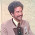## 2018-07-02

### How Tall is that Humanoid?

Let's look at all the height-values explicitly given for monsters in OD&D Vol-2 (there aren't that many; mostly just giant types) for some kind of pattern.

We assume the following: Hit dice are related to strength. And strength is related to cross-sectional area of the body. (This is certainly true for simple physical systems, where compressive strength is a function of area. It is broadly true in muscle physiology. However, it varies between individuals of a species based on gender, training, etc.)

Below we map the hit dice of various OD&D Vol-2 creatures to the square of their stated height (i.e., something proportional to their cross-sectional area). We include Men as a known quantity from the real world (taking 5' 8" as the mean height of medieval men). Also, we exclude Fire Giants as a rather obvious outlier (they are given proportions that are notably dwarfish, likely an allusion to the deformed god Hephaestus and many other mythological smiths/craftsmen).

Of course, we do not expect that these game values were set up with any kind of mechanical system in mind (on the other hand, it's pretty intuitive that strength and size should be increasing together). That said, we do find that a regression on these values gives a 96% coefficient of determination; a good match.

So if we want to invert this and use it as a rule-of-thumb, given that hit dice are given for every monster and height is usually not, we could say roughly that height (in feet) = √(27.71 HD) = 5.3 √HD5√HD.

Let's spot-check a few simple values. For Men (HD 1), our rough estimate would give 5√1 = 5×1 = 5 feet. For Ogres, we get 5√4 = 5×2 =10 feet (whereas the book says they "range from 7 to 10 feet in height"). For Stone Giants, we get 5√9 = 5×3 =15 feet (precisely the book figure). And so forth; it seems to work pretty well for creatures with man-like proportions.

For more serpentine creatures (like Tolkien-style dragons), we might say they have double the length given by this formula. In fact, we can check against the only other creature with a stated length in Vol-2: the Purple Worm, of which it is said that "some reach a length of 50 feet". Assume this is for the largest of the species, i.e., 6 pips per hit die, so 15 × 6 = 90 hit points. This equates to standard monster hit dice of 90 / 3.5 = 25. And our rule-of-thumb would estimate the corresponding serpent length as 2×5√25 = 2×5×5 = 50 feet. Well, isn't that nice.

Bonus: How Heavy is that Humanoid? This happened to come up (half-jokingly) in our game last weekend. Spring-boarding off the above, this needs to be proportional to the cube of the square-root of hit dice, that is, HD^(3/2) = HD^1.5. For the coefficient (multiplier), we back-calculate from information on the Corpulence Index (CI): We find that one can very roughly use weight (in pounds) = 100 × HD^1.5. For a somewhat better estimate, use 120 as the multiplier. For a value in stone-weight, use 8 as the multiplier. (Compare to "How Heavy is My Giant?" in Dragon #13, which relates height to weight but not hit dice.)

1.Lets take it for a spin
Goblin: HD 1(-1); 5x√1 = 5; minus 1= 4'
Kobold: HD 1/2; 5x√.5 = 3.5'
Gargoyle: HD 4; 5x√4 = 10' (wingspan?)

Works for me.

1.Excellent!

2.Possibly of related interest: the 10' pole in the picture on the back of In Search of the Unknown can be used to figure the heights of the characters shown:

1.OMG I love that! :-)

3.The human height also works out great if you take 5'8" to be the height of a 1+1 (1.3) HD fighter.

1.Good observation.